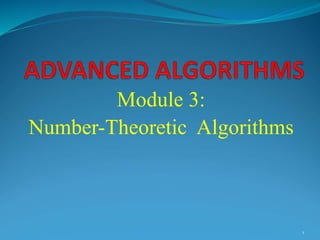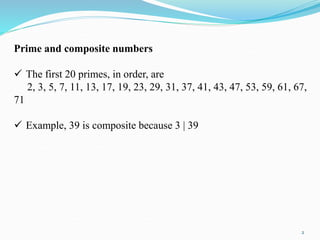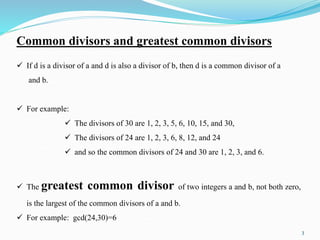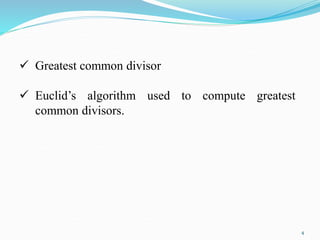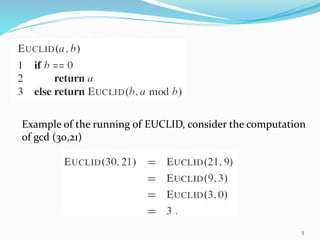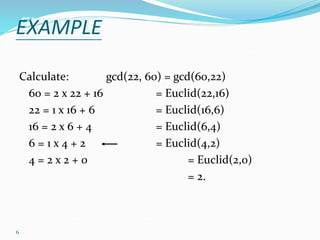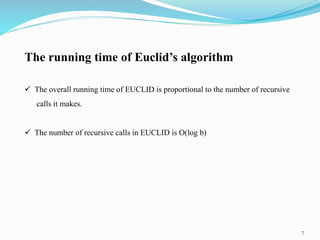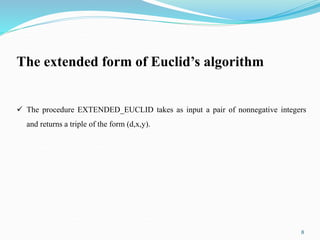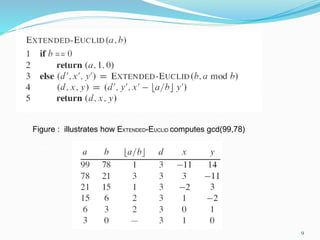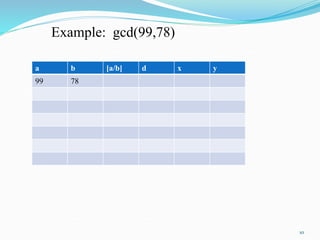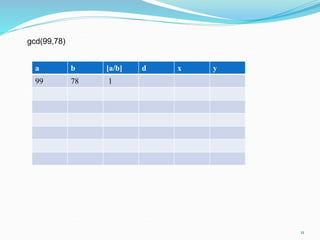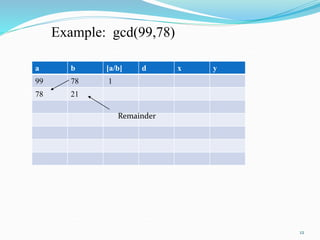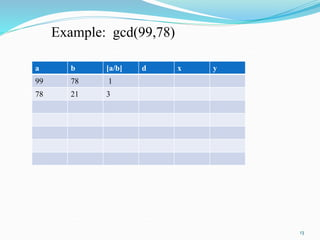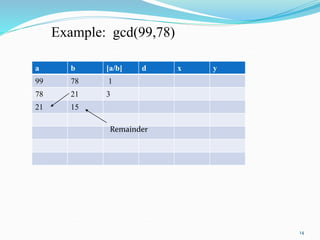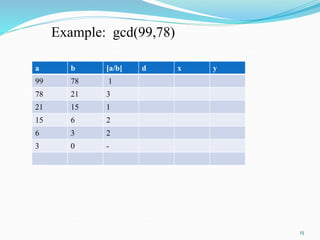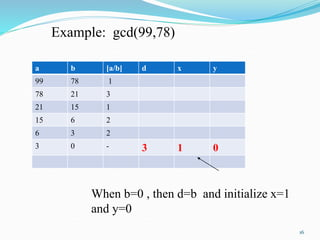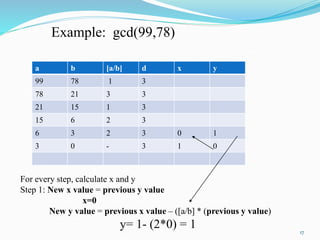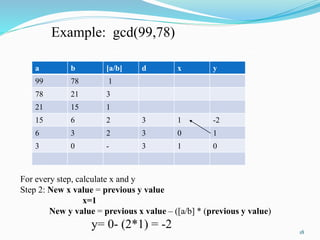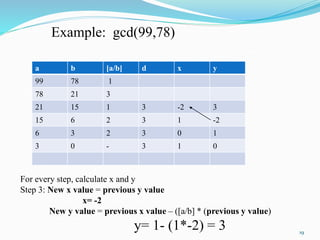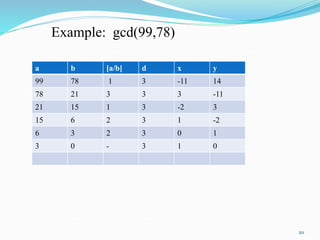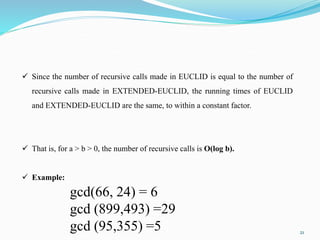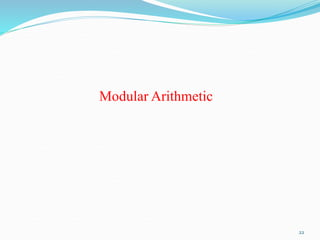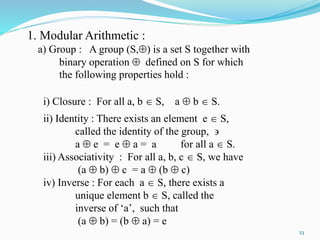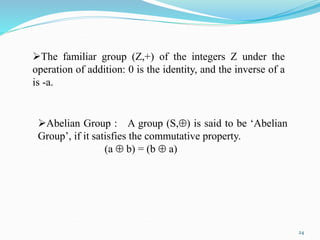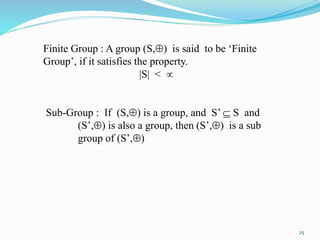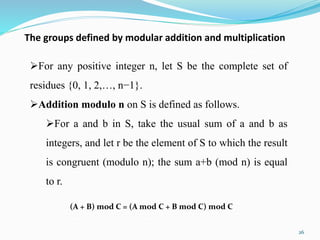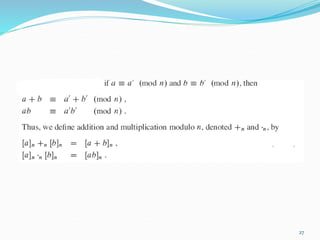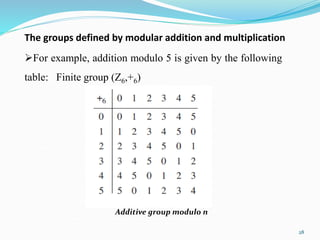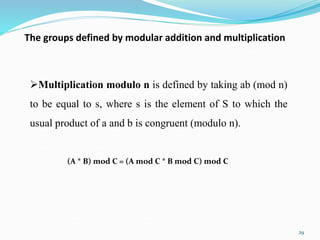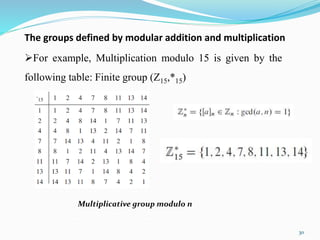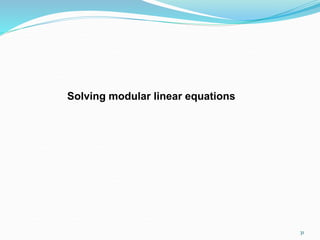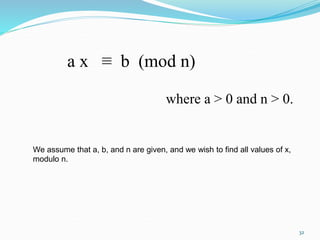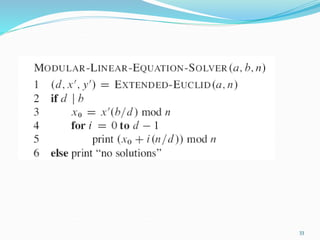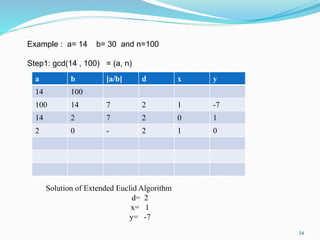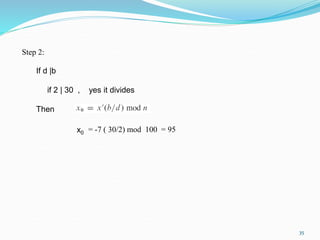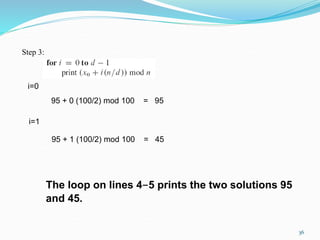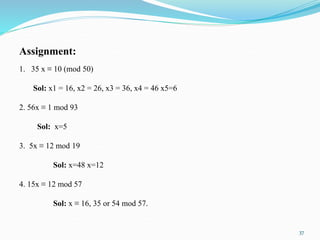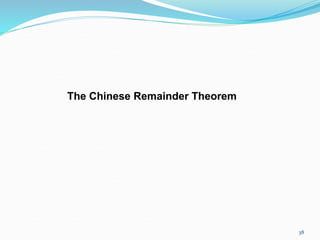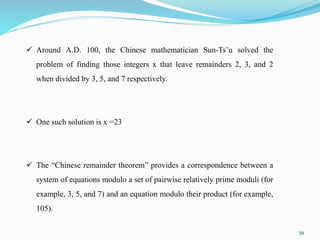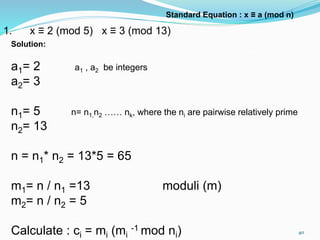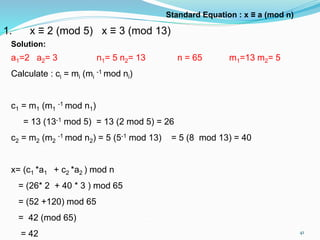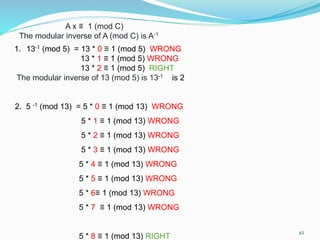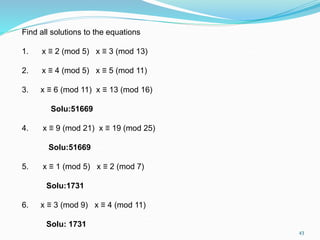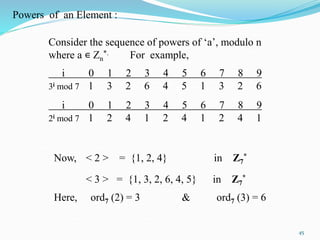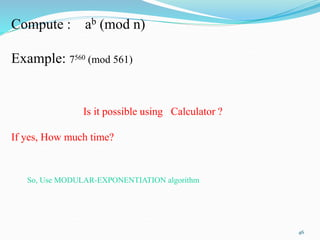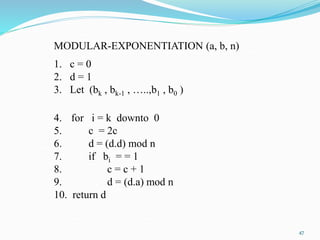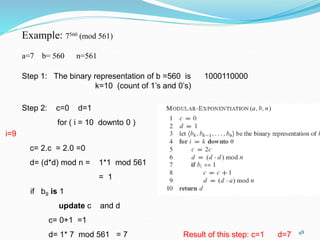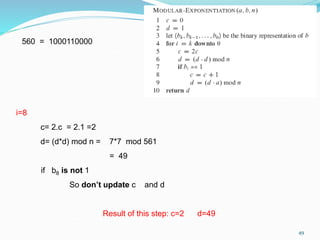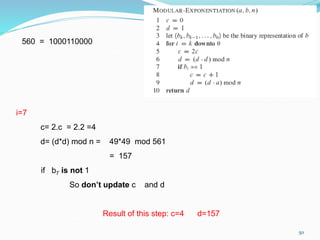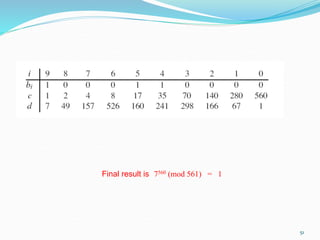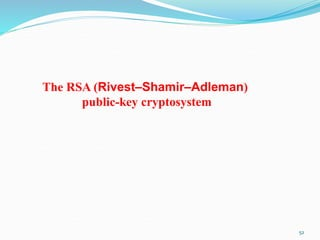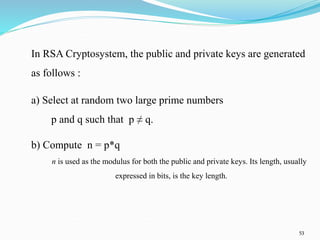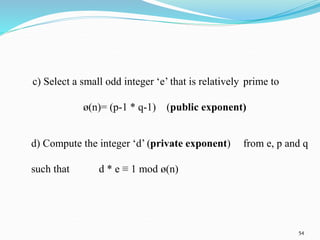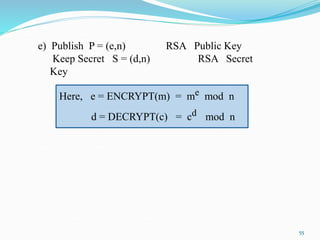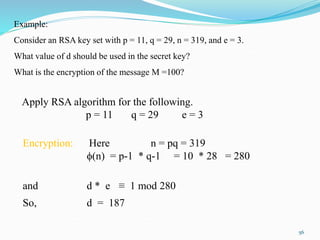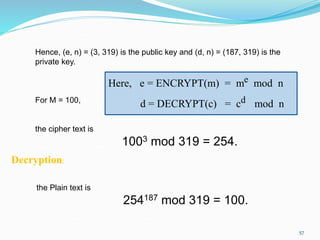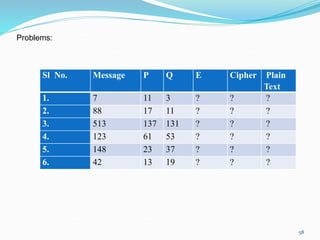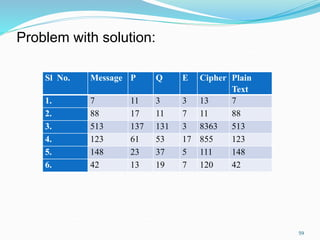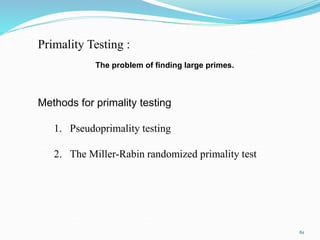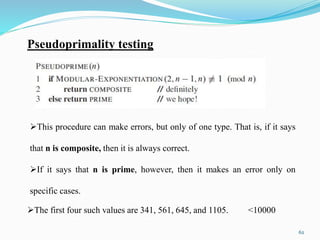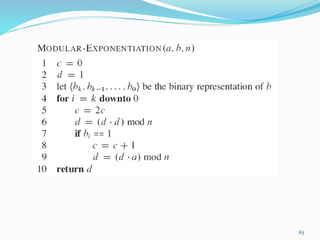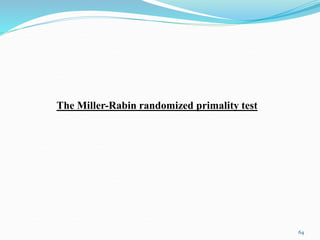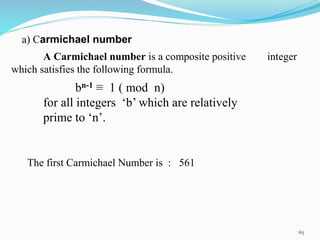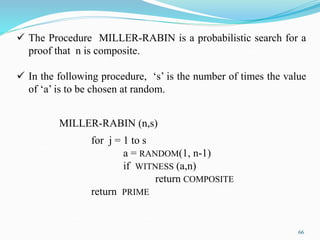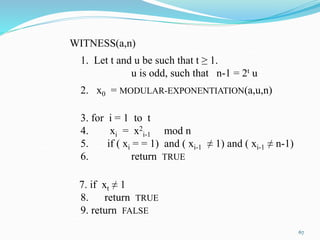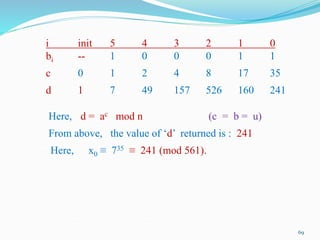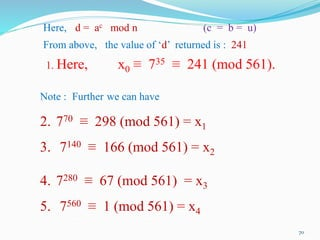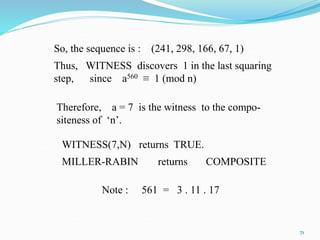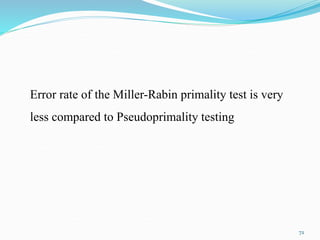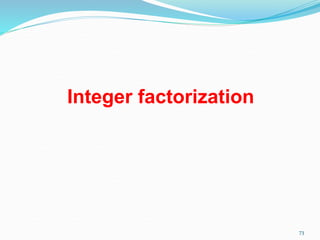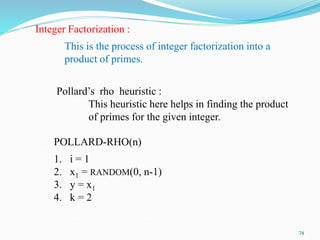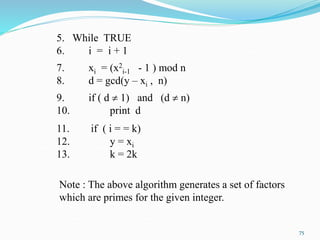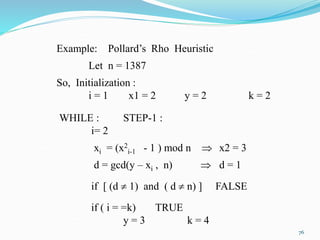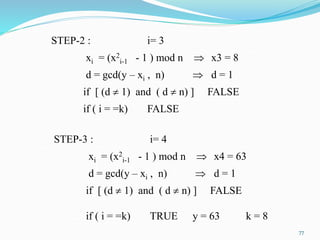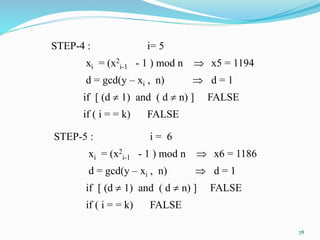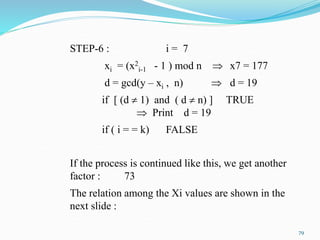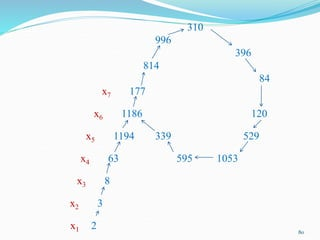1 of 80

1. Module 3: Number-Theoretic Algorithms 1
2. 2 Prime and composite numbers  The first 20 primes, in order, are 2, 3, 5, 7, 11, 13, 17, 19, 23, 29, 31, 37, 41, 43, 47, 53, 59, 61, 67, 71  Example, 39 is composite because 3 | 39
3. 3 Common divisors and greatest common divisors  If d is a divisor of a and d is also a divisor of b, then d is a common divisor of a and b.  For example:  The divisors of 30 are 1, 2, 3, 5, 6, 10, 15, and 30,  The divisors of 24 are 1, 2, 3, 6, 8, 12, and 24  and so the common divisors of 24 and 30 are 1, 2, 3, and 6.  The greatest common divisor of two integers a and b, not both zero, is the largest of the common divisors of a and b.  For example: gcd(24,30)=6
4. 4  Greatest common divisor  Euclid’s algorithm used to compute greatest common divisors.
5. 5 Example of the running of EUCLID, consider the computation of gcd (30,21)
6. 6 EXAMPLE Calculate: gcd(22, 60) = gcd(60,22) 60 = 2 x 22 + 16 = Euclid(22,16) 22 = 1 x 16 + 6 = Euclid(16,6) 16 = 2 x 6 + 4 = Euclid(6,4) 6 = 1 x 4 + 2 = Euclid(4,2) 4 = 2 x 2 + 0 = Euclid(2,0) = 2.
7. 7 The running time of Euclid’s algorithm  The overall running time of EUCLID is proportional to the number of recursive calls it makes.  The number of recursive calls in EUCLID is O(log b)
8. 8 The extended form of Euclid’s algorithm  The procedure EXTENDED_EUCLID takes as input a pair of nonnegative integers and returns a triple of the form (d,x,y).
9. 9 Figure : illustrates how EXTENDED-EUCLID computes gcd(99,78)
10. 10 a b [a/b] d x y 99 78 Example: gcd(99,78)
11. 11 a b [a/b] d x y 99 78 1 gcd(99,78)
12. 12 a b [a/b] d x y 99 78 1 78 21 Remainder Example: gcd(99,78)
13. 13 a b [a/b] d x y 99 78 1 78 21 3 Example: gcd(99,78)
14. 14 a b [a/b] d x y 99 78 1 78 21 3 21 15 Remainder Example: gcd(99,78)
15. 15 a b [a/b] d x y 99 78 1 78 21 3 21 15 1 15 6 2 6 3 2 3 0 - Example: gcd(99,78)
16. 16 a b [a/b] d x y 99 78 1 78 21 3 21 15 1 15 6 2 6 3 2 3 0 - 3 1 0 Example: gcd(99,78) When b=0 , then d=b and initialize x=1 and y=0
17. 17 a b [a/b] d x y 99 78 1 3 78 21 3 3 21 15 1 3 15 6 2 3 6 3 2 3 0 1 3 0 - 3 1 0 Example: gcd(99,78) For every step, calculate x and y Step 1: New x value = previous y value x=0 New y value = previous x value – ([a/b] * (previous y value) y= 1- (2*0) = 1
18. 18 a b [a/b] d x y 99 78 1 78 21 3 21 15 1 15 6 2 3 1 -2 6 3 2 3 0 1 3 0 - 3 1 0 For every step, calculate x and y Step 2: New x value = previous y value x=1 New y value = previous x value – ([a/b] * (previous y value) y= 0- (2*1) = -2 Example: gcd(99,78)
19. 19 a b [a/b] d x y 99 78 1 78 21 3 21 15 1 3 -2 3 15 6 2 3 1 -2 6 3 2 3 0 1 3 0 - 3 1 0 For every step, calculate x and y Step 3: New x value = previous y value x= -2 New y value = previous x value – ([a/b] * (previous y value) y= 1- (1*-2) = 3 Example: gcd(99,78)
20. 20 a b [a/b] d x y 99 78 1 3 -11 14 78 21 3 3 3 -11 21 15 1 3 -2 3 15 6 2 3 1 -2 6 3 2 3 0 1 3 0 - 3 1 0 Example: gcd(99,78)
21. 21  Since the number of recursive calls made in EUCLID is equal to the number of recursive calls made in EXTENDED-EUCLID, the running times of EUCLID and EXTENDED-EUCLID are the same, to within a constant factor.  That is, for a > b > 0, the number of recursive calls is O(log b).  Example: gcd(66, 24) = 6 gcd (899,493) =29 gcd (95,355) =5
22. 22 Modular Arithmetic
23. 23 1. Modular Arithmetic : a) Group : A group (S,) is a set S together with binary operation  defined on S for which the following properties hold : i) Closure : For all a, b  S, a  b  S. ii) Identity : There exists an element e  S, called the identity of the group,  a  e = e  a = a for all a  S. iii) Associativity : For all a, b, c  S, we have (a  b)  c = a  (b  c) iv) Inverse : For each a  S, there exists a unique element b  S, called the inverse of ‘a’, such that (a  b) = (b  a) = e
24. 24 The familiar group (Z,+) of the integers Z under the operation of addition: 0 is the identity, and the inverse of a is -a. Abelian Group : A group (S,) is said to be ‘Abelian Group’, if it satisfies the commutative property. (a  b) = (b  a)
25. 25 Finite Group : A group (S,) is said to be ‘Finite Group’, if it satisfies the property. |S| <  Sub-Group : If (S,) is a group, and S’  S and (S’,) is also a group, then (S’,) is a sub group of (S’,)
26. 26 The groups defined by modular addition and multiplication For any positive integer n, let S be the complete set of residues {0, 1, 2,…, n−1}. Addition modulo n on S is defined as follows. For a and b in S, take the usual sum of a and b as integers, and let r be the element of S to which the result is congruent (modulo n); the sum a+b (mod n) is equal to r. (A + B) mod C = (A mod C + B mod C) mod C
27. 27
28. 28 The groups defined by modular addition and multiplication For example, addition modulo 5 is given by the following table: Finite group (Z6,+6) Additive group modulo n
29. 29 The groups defined by modular addition and multiplication Multiplication modulo n is defined by taking ab (mod n) to be equal to s, where s is the element of S to which the usual product of a and b is congruent (modulo n). (A * B) mod C = (A mod C * B mod C) mod C
30. 30 The groups defined by modular addition and multiplication For example, Multiplication modulo 15 is given by the following table: Finite group (Z15,*15) Multiplicative group modulo n
31. 31 Solving modular linear equations
32. 32 a x ≡ b (mod n) where a > 0 and n > 0. We assume that a, b, and n are given, and we wish to find all values of x, modulo n.
33. 33
34. 34 Example : a= 14 b= 30 and n=100 Step1: gcd(14 , 100) = (a, n) a b [a/b] d x y 14 100 100 14 7 2 1 -7 14 2 7 2 0 1 2 0 - 2 1 0 Solution of Extended Euclid Algorithm d= 2 x= 1 y= -7
35. 35 Step 2: If d |b if 2 | 30 , yes it divides Then x0 = -7 ( 30/2) mod 100 = 95
36. 36 Step 3: i=0 95 + 0 (100/2) mod 100 = 95 i=1 95 + 1 (100/2) mod 100 = 45 The loop on lines 4-5 prints the two solutions 95 and 45.
37. 37 Assignment: 1. 35 x ≡ 10 (mod 50) Sol: x1 = 16, x2 = 26, x3 = 36, x4 = 46 x5=6 2. 56x ≡ 1 mod 93 Sol: x=5 3. 5x ≡ 12 mod 19 Sol: x=48 x=12 4. 15x ≡ 12 mod 57 Sol: x ≡ 16, 35 or 54 mod 57.
38. 38 The Chinese Remainder Theorem
39. 39  Around A.D. 100, the Chinese mathematician Sun-Ts˘u solved the problem of finding those integers x that leave remainders 2, 3, and 2 when divided by 3, 5, and 7 respectively.  One such solution is x =23  The “Chinese remainder theorem” provides a correspondence between a system of equations modulo a set of pairwise relatively prime moduli (for example, 3, 5, and 7) and an equation modulo their product (for example, 105).
40. 40 1. x ≡ 2 (mod 5) x ≡ 3 (mod 13) Standard Equation : x ≡ a (mod n) Solution: a1= 2 a1 , a2 be integers a2= 3 n1= 5 n= n1,n2 …… nk, where the ni are pairwise relatively prime n2= 13 n = n1* n2 = 13*5 = 65 m1= n / n1 =13 moduli (m) m2= n / n2 = 5 Calculate : ci = mi (mi -1 mod ni)
41. 41 1. x ≡ 2 (mod 5) x ≡ 3 (mod 13) Standard Equation : x ≡ a (mod n) Solution: a1=2 a2= 3 n1= 5 n2= 13 n = 65 m1=13 m2= 5 Calculate : ci = mi (mi -1 mod ni) c1 = m1 (m1 -1 mod n1) = 13 (13-1 mod 5) = 13 (2 mod 5) = 26 c2 = m2 (m2 -1 mod n2) = 5 (5-1 mod 13) = 5 (8 mod 13) = 40 x= (c1 *a1 + c2 *a2 ) mod n = (26* 2 + 40 * 3 ) mod 65 = (52 +120) mod 65 = 42 (mod 65) = 42
42. 42 1. 13-1 (mod 5) = 13 * 0 ≡ 1 (mod 5) WRONG 13 * 1 ≡ 1 (mod 5) WRONG 13 * 2 ≡ 1 (mod 5) RIGHT The modular inverse of 13 (mod 5) is 13-1 is 2 A x ≡ 1 (mod C) The modular inverse of A (mod C) is A-1 2. 5 -1 (mod 13) = 5 * 0 ≡ 1 (mod 13) WRONG 5 * 1 ≡ 1 (mod 13) WRONG 5 * 2 ≡ 1 (mod 13) WRONG 5 * 3 ≡ 1 (mod 13) WRONG 5 * 4 ≡ 1 (mod 13) WRONG 5 * 5 ≡ 1 (mod 13) WRONG 5 * 6≡ 1 (mod 13) WRONG 5 * 7 ≡ 1 (mod 13) WRONG 5 * 8 ≡ 1 (mod 13) RIGHT
43. 43 Find all solutions to the equations 1. x ≡ 2 (mod 5) x ≡ 3 (mod 13) 2. x ≡ 4 (mod 5) x ≡ 5 (mod 11) 3. x ≡ 6 (mod 11) x ≡ 13 (mod 16) Solu:51669 4. x ≡ 9 (mod 21) x ≡ 19 (mod 25) Solu:51669 5. x ≡ 1 (mod 5) x ≡ 2 (mod 7) Solu:1731 6. x ≡ 3 (mod 9) x ≡ 4 (mod 11) Solu: 1731
44. 44 Powers of an Element
45. 45 Consider the sequence of powers of ‘a’, modulo n where a ∊ Zn *. For example, i 0 1 2 3 4 5 6 7 8 9 3i mod 7 1 3 2 6 4 5 1 3 2 6 i 0 1 2 3 4 5 6 7 8 9 2i mod 7 1 2 4 1 2 4 1 2 4 1 Now, < 2 > = {1, 2, 4} in Z7 * < 3 > = {1, 3, 2, 6, 4, 5} in Z7 * Here, ord7 (2) = 3 & ord7 (3) = 6 Powers of an Element :
46. 46 Compute : ab (mod n) Example: 7560 (mod 561) Is it possible using Calculator ? If yes, How much time? So, Use MODULAR-EXPONENTIATION algorithm
47. 47 MODULAR-EXPONENTIATION (a, b, n) 1. c = 0 2. d = 1 3. Let (bk , bk-1 , …..,b1 , b0 ) 4. for i = k downto 0 5. c = 2c 6. d = (d.d) mod n 7. if bi = = 1 8. c = c + 1 9. d = (d.a) mod n 10. return d
48. 48 Example: 7560 (mod 561) a=7 b= 560 n=561 Step 1: The binary representation of b =560 is 1000110000 k=10 (count of 1’s and 0’s) Step 2: c=0 d=1 for ( i = 10 downto 0 ) i=9 c= 2.c = 2.0 =0 d= (d*d) mod n = 1*1 mod 561 = 1 if b9 is 1 update c and d c= 0+1 =1 d= 1* 7 mod 561 = 7 Result of this step: c=1 d=7
49. 49 i=8 c= 2.c = 2.1 =2 d= (d*d) mod n = 7*7 mod 561 = 49 if b8 is not 1 So don’t update c and d Result of this step: c=2 d=49 560 = 1000110000
50. 50 i=7 c= 2.c = 2.2 =4 d= (d*d) mod n = 49*49 mod 561 = 157 if b7 is not 1 So don’t update c and d Result of this step: c=4 d=157 560 = 1000110000
51. 51 Final result is 7560 (mod 561) = 1
52. 52 The RSA (Rivest–Shamir–Adleman) public-key cryptosystem
53. 53 In RSA Cryptosystem, the public and private keys are generated as follows : a) Select at random two large prime numbers p and q such that p ≠ q. b) Compute n = p*q n is used as the modulus for both the public and private keys. Its length, usually expressed in bits, is the key length.
54. 54 c) Select a small odd integer ‘e’ that is relatively prime to ø(n)= (p-1 * q-1) (public exponent) d) Compute the integer ‘d’ (private exponent) from e, p and q such that d * e ≡ 1 mod ø(n)
55. 55 e) Publish P = (e,n) RSA Public Key Keep Secret S = (d,n) RSA Secret Key Here, e = ENCRYPT(m) = me mod n d = DECRYPT(c) = cd mod n
56. 56 Apply RSA algorithm for the following. p = 11 q = 29 e = 3 Encryption: Here n = pq = 319 (n) = p-1 * q-1 = 10 * 28 = 280 and d * e ≡ 1 mod 280 So, d = 187 Example: Consider an RSA key set with p = 11, q = 29, n = 319, and e = 3. What value of d should be used in the secret key? What is the encryption of the message M =100?
57. 57 Hence, (e, n) = (3, 319) is the public key and (d, n) = (187, 319) is the private key. For M = 100, the cipher text is 1003 mod 319 = 254. Here, e = ENCRYPT(m) = me mod n d = DECRYPT(c) = cd mod n Decryption: the Plain text is 254187 mod 319 = 100.
58. 58 Problems: Sl No. Message P Q E Cipher Plain Text 1. 7 11 3 ? ? ? 2. 88 17 11 ? ? ? 3. 513 137 131 ? ? ? 4. 123 61 53 ? ? ? 5. 148 23 37 ? ? ? 6. 42 13 19 ? ? ?
59. 59 Problem with solution: Sl No. Message P Q E Cipher Plain Text 1. 7 11 3 3 13 7 2. 88 17 11 7 11 88 3. 513 137 131 3 8363 513 4. 123 61 53 17 855 123 5. 148 23 37 5 111 148 6. 42 13 19 7 120 42
60. 60 Primality Testing
61. 61 Primality Testing : Methods for primality testing 1. Pseudoprimality testing 2. The Miller-Rabin randomized primality test The problem of finding large primes.
62. 62 Pseudoprimality testing This procedure can make errors, but only of one type. That is, if it says that n is composite, then it is always correct. If it says that n is prime, however, then it makes an error only on specific cases. The first four such values are 341, 561, 645, and 1105. <10000
63. 63
64. 64 The Miller-Rabin randomized primality test
65. 65 a) Carmichael number A Carmichael number is a composite positive integer which satisfies the following formula. bn-1 ≡ 1 ( mod n) for all integers ‘b’ which are relatively prime to ‘n’. The first Carmichael Number is : 561
66. 66 MILLER-RABIN (n,s) for j = 1 to s a = RANDOM(1, n-1) if WITNESS (a,n) return COMPOSITE return PRIME  The Procedure MILLER-RABIN is a probabilistic search for a proof that n is composite.  In the following procedure, ‘s’ is the number of times the value of ‘a’ is to be chosen at random.
67. 67 WITNESS(a,n) 1. Let t and u be such that t ≥ 1. u is odd, such that n-1 = 2t u 2. x0 = MODULAR-EXPONENTIATION(a,u,n) 3. for i = 1 to t 4. xi = x2 i-1 mod n 5. if ( xi = = 1) and ( xi-1 ≠ 1) and ( xi-1 ≠ n-1) 6. return TRUE 7. if xt ≠ 1 8. return TRUE 9. return FALSE
68. 68 Example: Let ‘n’ be a carmichael number. n = 561 So, here n – 1 = 560 If n – 1 is written in the form of n-1 = 2t u, then t = 4 and u = 35 Let the value of ‘a’ is chosen from the algorithm as : 7 From the WITNESS algorithm, find the value of x0. Here, call the MODULAR_EXPONENTIATION(a,u,n) where a = 7 & u = 35 & n = 561
69. 69 Here, d = ac mod n (c = b = u) From above, the value of ‘d’ returned is : 241 Here, x0 ≡ 735 ≡ 241 (mod 561). i init 5 4 3 2 1 0 bi -- 1 0 0 0 1 1 c 0 1 2 4 8 17 35 d 1 7 49 157 526 160 241
70. 70 Here, d = ac mod n (c = b = u) From above, the value of ‘d’ returned is : 241 1. Here, x0 ≡ 735 ≡ 241 (mod 561). Note : Further we can have 2. 770 ≡ 298 (mod 561) = x1 3. 7140 ≡ 166 (mod 561) = x2 4. 7280 ≡ 67 (mod 561) = x3 5. 7560 ≡ 1 (mod 561) = x4
71. 71 So, the sequence is : (241, 298, 166, 67, 1) Thus, WITNESS discovers 1 in the last squaring step, since a560 ≡ 1 (mod n) Therefore, a = 7 is the witness to the compo- siteness of ‘n’. WITNESS(7,N) returns TRUE. MILLER-RABIN returns COMPOSITE Note : 561 = 3 . 11 . 17
72. 72 Error rate of the Miller-Rabin primality test is very less compared to Pseudoprimality testing
73. 73 Integer factorization
74. 74 Integer Factorization : This is the process of integer factorization into a product of primes. Pollard’s rho heuristic : This heuristic here helps in finding the product of primes for the given integer. POLLARD-RHO(n) 1. i = 1 2. x1 = RANDOM(0, n-1) 3. y = x1 4. k = 2
75. 75 5. While TRUE 6. i = i + 1 7. xi = (x2 i-1 - 1 ) mod n 8. d = gcd(y – xi , n) 9. if ( d  1) and (d  n) 10. print d 11. if ( i = = k) 12. y = xi 13. k = 2k Note : The above algorithm generates a set of factors which are primes for the given integer.
76. 76 Example: Pollard’s Rho Heuristic Let n = 1387 So, Initialization : i = 1 x1 = 2 y = 2 k = 2 WHILE : STEP-1 : i= 2 xi = (x2 i-1 - 1 ) mod n  x2 = 3 d = gcd(y – xi , n)  d = 1 if [ (d  1) and ( d  n) ] FALSE if ( i = =k) TRUE y = 3 k = 4
77. 77 STEP-2 : i= 3 xi = (x2 i-1 - 1 ) mod n  x3 = 8 d = gcd(y – xi , n)  d = 1 if [ (d  1) and ( d  n) ] FALSE if ( i = =k) FALSE STEP-3 : i= 4 xi = (x2 i-1 - 1 ) mod n  x4 = 63 d = gcd(y – xi , n)  d = 1 if [ (d  1) and ( d  n) ] FALSE if ( i = =k) TRUE y = 63 k = 8
78. 78 STEP-4 : i= 5 xi = (x2 i-1 - 1 ) mod n  x5 = 1194 d = gcd(y – xi , n)  d = 1 if [ (d  1) and ( d  n) ] FALSE if ( i = = k) FALSE STEP-5 : i = 6 xi = (x2 i-1 - 1 ) mod n  x6 = 1186 d = gcd(y – xi , n)  d = 1 if [ (d  1) and ( d  n) ] FALSE if ( i = = k) FALSE
79. 79 STEP-6 : i = 7 xi = (x2 i-1 - 1 ) mod n  x7 = 177 d = gcd(y – xi , n)  d = 19 if [ (d  1) and ( d  n) ] TRUE  Print d = 19 if ( i = = k) FALSE If the process is continued like this, we get another factor : 73 The relation among the Xi values are shown in the next slide :
80. 80 310 996 396 814 84 x7 177 x6 1186 120 x5 1194 339 529 x4 63 595 1053 x3 8 x2 3 x1 2